Courses

# Binary Heaps MCQ - 1

## 20 Questions MCQ Test RRB JE for Computer Science Engineering | Binary Heaps MCQ - 1

Description
This mock test of Binary Heaps MCQ - 1 for Computer Science Engineering (CSE) helps you for every Computer Science Engineering (CSE) entrance exam. This contains 20 Multiple Choice Questions for Computer Science Engineering (CSE) Binary Heaps MCQ - 1 (mcq) to study with solutions a complete question bank. The solved questions answers in this Binary Heaps MCQ - 1 quiz give you a good mix of easy questions and tough questions. Computer Science Engineering (CSE) students definitely take this Binary Heaps MCQ - 1 exercise for a better result in the exam. You can find other Binary Heaps MCQ - 1 extra questions, long questions & short questions for Computer Science Engineering (CSE) on EduRev as well by searching above.
QUESTION: 1

### What is the time complexity of Build Heap operation. Build Heap is used to build a max(or min) binary heap from a given array. Build Heap is used in Heap Sort as a first step for sorting.

Solution:

Following is algorithm for building a Heap of an input array A.

BUILD-HEAP(A)
heapsize := size(A);
for i := floor(heapsize/2) downto 1
do HEAPIFY(A, i);
end for
END

Although the worst case complexity looks like O(nLogn), upper bound of time complexity is O(n).

QUESTION: 2

### Suppose we are sorting an array of eight integers using heapsort, and we have just finished some heapify (either maxheapify or minheapify) operations. The array now looks like this: 16 14 15 10 12 27 28 How many heapify operations have been performed on root of heap?

Solution:

In Heapsort, we first build a heap, then we do following operations till the heap size becomes 1. a) Swap the root with last element b) Call heapify for root c) reduce the heap size by 1. In this question, it is given that heapify has been called few times and we see that last two elements in given array are the 2 maximum elements in array. So situation is clear, it is maxheapify whic has been called 2 times.

QUESTION: 3

### A max-heap is a heap where the value of each parent is greater than or equal to the values of its children. Which of the following is a max-heap? (GATE CS 2011)

Solution:
QUESTION: 4

A 3-ary max heap is like a binary max heap, but instead of 2 children, nodes have 3 children. A 3-ary heap can be represented by an array as follows: The root is stored in the first location, a, nodes in the next level, from left to right, is stored from a to a. The nodes from the second level of the tree from left to right are stored from a location onward. An item x can be inserted into a 3-ary heap containing n items by placing x in the location a[n] and pushing it up the tree to satisfy the heap property. Which one of the following is a valid sequence of elements in an array representing 3-ary max heap?

Solution:

Following 3-ary Max Heap can be constructed from sequence given option (D)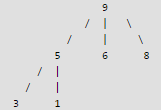QUESTION: 5

Suppose the elements 7, 2, 10 and 4 are inserted, in that order, into the valid 3- ary max heap found in the above question, Which one of the following is the sequence of items in the array representing the resultant heap?

Solution:

After insertion of 7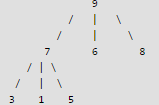After insertion of 2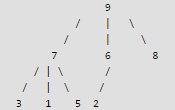After insertion of 10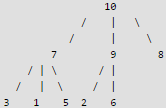After insertion of 4QUESTION: 6

Consider a binary max-heap implemented using an array. Which one of the following array represents a binary max-heap?

Solution:

A tree is max-heap if data at every node in the tree is greater than or equal to it’s children’ s data. In array representation of heap tree, a node at index i has its left child at index 2i + 1 and right child at index 2i + 2.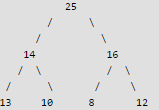QUESTION: 7

What is the content of the array after two delete operations on the correct answer to the previous question?

Solution:

For Heap trees, deletion of a node includes following two operations. 1) Replace the root with last element on the last level. 2) Starting from root, heapify the complete tree from top to bottom.. Let us delete the two nodes one by one: 1) Deletion of 25: Replace 25 with 12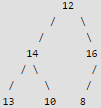13 10 8

Since heap property is violated for root (16 is greater than 12), make 16 as root of the tree.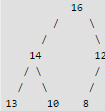2) Deletion of 16: Replace 16 with 8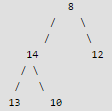Heapify from root to bottom.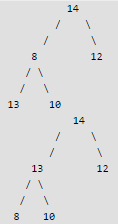QUESTION: 8

We have a binary heap on n elements and wish to insert n more elements (not necessarily one after another) into this heap. The total time required for this is

Solution:

We can reduce the problem to Build Heap for 2n elements. Time taken for build heap is O(n)

QUESTION: 9

In a min-heap with n elements with the smallest element at the root, the 7th smallest element can be found in time.

The question was not clear in original GATE exam. For clarity, assume that there are no duplicates in Min-Heap and accessing heap elements below root is allowed.

Solution:

The 7th smallest element must be in first 7 levels. Total number of nodes in any Binary Heap in first 7 levels is at most 1 + 2 + 4 + 8 + 16 + 32 + 64 which is a constant. Therefore we can always find 7th smallest element in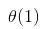time. If Min-Heap is allowed to have duplicates, then time complexity becomes Θ(Log n). Also, if Min-Heap doesn't allow directly accessing elements below root and supports only extract-min() operation, then also time complexity becomes Θ(Log n).

QUESTION: 10

In a binary max heap containing n numbers, the smallest element can be found in time

Solution:

In a max heap, the smallest element is always present at a leaf node. So we need to check for all leaf nodes for the minimum value. Worst case complexity will be O(n)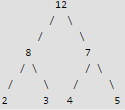QUESTION: 11

The elements 32, 15, 20, 30, 12, 25, 16 are inserted one by one in the given order into a Max Heap. The resultant Max Heap is.

Solution:

32, 15, 20, 30, 12, 25, 16
After insertion of 32, 15 and 20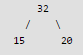After insertion of 30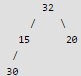Max Heap property is violated, so 30 is swapped with 15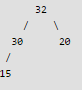After insertion of 12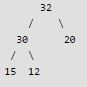After insertion of 25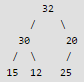Max Heap property is violated, so 25 is swapped with 20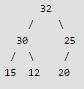After insertion of 16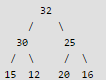QUESTION: 12

Given two max heaps of size n each, what is the minimum possible time complexity to make a one max-heap of size from elements of two max heaps?

Solution:

We can build a heap of 2n elements in O(n) time. Following are the steps. Create an array of size 2n and copy elements of both heaps to this array. Call build heap for the array of size 2n. Build heap operation takes O(n) time.

QUESTION: 13

A priority queue is implemented as a Max-Heap. Initially, it has 5 elements. The level-order traversal of the heap is: 10, 8, 5, 3, 2. Two new elements 1 and 7 are inserted into the heap in that order. The level-order traversal of the heap after the insertion of the elements is:

Solution:

Initially heap has 10, 8, 5, 3, 2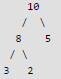After insertion of 1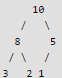No need to heapify as 5 is greater than 1.

After insertion of 7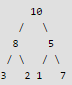Heapify 5 as 7 is greater than 5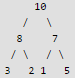No need to heapify any further as 10 is greater than 7

QUESTION: 14

Which of the following Binary Min Heap operation has the highest time complexity?

Solution:

The merge operation takes O(n) time, all other operations given in question take O(Logn) time. The Binomial and Fibonacci Heaps do merge in better time complexity.

QUESTION: 15

Consider any array representation of an n element binary heap where the elements are stored from index 1 to index n of the array. For the element stored at index i of the array (i <= n), the index of the parent is

Solution:

Binary heaps can be represented using arrays: storing elements in an array and using their relative positions within the array to represent child-parent relationships. For the binary heap element stored at index i of the array, Parent Node will be at index: floor(i/2) Left Child will be at index: 2i Right child will be at index: 2*i + 1

QUESTION: 16

Consider a max heap, represented by the array: 40, 30, 20, 10, 15, 16, 17, 8, 4. Now consider that a value 35 is inserted into this heap. After insertion, the new heap is

Solution:

The array 40, 30, 20, 10, 15, 16, 17, 8, 4 represents following heap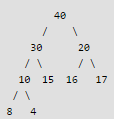After insertion of 35, we get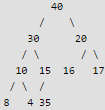After swapping 35 with 15 and swapping 35 again with 30, we get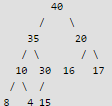QUESTION: 17

Consider the following array of elements. 〈89, 19, 50, 17, 12, 15, 2, 5, 7, 11, 6, 9, 100〉. The minimum number of interchanges needed to convert it into a max-heap is

Solution:

〈89, 19, 50, 17, 12, 15, 2, 5, 7, 11, 6, 9, 100〉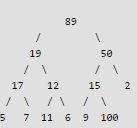Minimum number of swaps required to convert above tree to Max heap is 3. Below are 3 swap operations.
Swap 100 with 15
Swap 100 with 50
Swap 100 with 89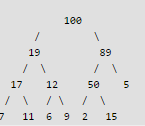QUESTION: 18

An operator delete(i) for a binary heap data structure is to be designed to delete the item in the i-th node. Assume that the heap is implemented in an array and i refers to the i-th index of the array. If the heap tree has depth d (number of edges on the path from the root to the farthest leaf), then what is the time complexity to re-fix the heap efficiently after the removal of the element?

Solution:

For this question, we have to slightly tweak the delete_min() operation of the heap data structure to implement the delete(i) operation. The idea is to empty the spot in the array at the index i (the position at which element is to be deleted) and replace it with the last leaf in the heap (remember heap is implemented as complete binary tree so you know the location of the last leaf), decrement the heap size and now starting from the current position (position that held the item we deleted), shift it up in case newly replaced item is greater than the parent of old item  (considering max-heap).  If it’s not greater than the parent, then percolate it down by comparing with the child’s value. The newly added item can percolate up/down a maximum of d times which is the depth of the heap data structure. Thus we can say that complexity of delete(i) would be O(d) but not O(1).

QUESTION: 19

A complete binary min-heap is made by including each integer in [1, 1023] exactly once. The depth of a node in the heap is the length of the path from the root of the heap to that node. Thus, the root is at depth 0. The maximum depth at which integer 9 can appear is _____________

Solution:

here node with integer 1 has to be at root only. Now for maximum depth of the tree the following arrangement can be taken. Take root as level 1. make node 2 at level 2 as a child node of node 1. make node 3 at level 3 as the child node of node 2. .. .. and so on for nodes 4,5,6,7 .. make node 8 at level 8 as the child node of node 7. make node 9 at level 9 as the child node of node 8. Putting other nodes properly, this arrangement of the the complete binary tree will follow the property of min heap. So total levels are 9. node 9 is at level 9 and depth of node 9 is 8 from the root.

QUESTION: 20

Which of the following sequences of array elements forms a heap?

Solution:

When they are asking for heap, by default it's max heap. Basic Requirement: Array representation of binary tree Starting from basics lets first understand heap trees We have 2 types of heap – Min heap and Max heap In Min heap the parent is always smaller than its children and in Max heap parent is always greater than its children.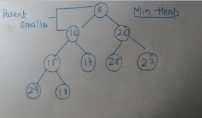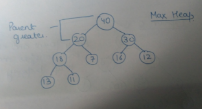Looking at the options we can tell that which tree is Max heap tree. Now consider each option one by one and draw a tree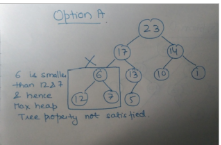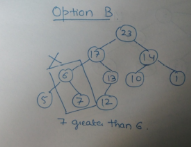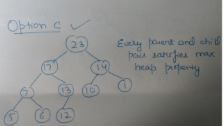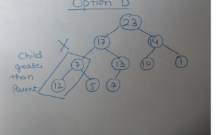From options it is clear that only option C satisfies the Max heap tree property.